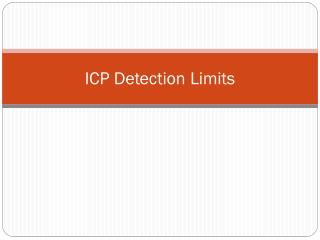Download PresentationICP Detection Limits

ICP Detection Limits - PowerPoint PPT Presentation

ICP Detection Limits. Detection Limit (DL) or Limit of Detection (LOD). The detection limit is the concentration that is obtained when the measured signal differs significantly from the background. Calculated by this equation for the ARCOS. C 1 = concentration of the high sampleI am the owner, or an agent authorized to act on behalf of the owner, of the copyrighted work described.
Download PresentationICP Detection Limits

Download Policy: Content on the Website is provided to you AS IS for your information and personal use and may not be sold / licensed / shared on other websites without getting consent from its author.While downloading, if for some reason you are not able to download a presentation, the publisher may have deleted the file from their server.

- - - - - - - - - - - - - - - - - - - - - - - - - - E N D - - - - - - - - - - - - - - - - - - - - - - - - - -
Presentation Transcript
1. ICP Detection Limits

2. Detection Limit (DL) or Limit of Detection (LOD) • The detection limit is the concentration that is obtained when the measured signal differs significantly from the background. • Calculated by this equation for the ARCOS. • C1 = concentration of the high sample • C0 = concentration of the blank • I1 = raw intensity of the high sample (cps) • I0 = raw intensity of the blank (cps) • 3 is a confidence factor • σ(sigma) = standard deviation from a number of measurements of the blank (cps)

3. Confidence Factor - Example • Black curve – probability density function for the normal distribution of blank measurements. • LOD (Limit of detection) – defined here as 3 times the standard deviation of the blank. • α – alpha error (probability of a false positive) is only 1%. • β – beta error (probability of a false negative) - there is a 50% chance that the sample could contain the element, but record it as less than the LOD. • LOQ (Limit of quantification) – defined here as 10 times the standard deviation - a minimal chance of a false negative or a false positive. Gives greater confidence that the reported values are actually quantifiable.

4. Example for P177.495 -using 2 measurements • C1 = 20 ppm • C2 = 0 ppm • I1 = 171777 cps • I0 = 320 cps OR or ~0.019 ppm

5. ARCOS - Summary • If the method only does one measurement - the DLs are not automatically calculated. • Input your confidence factor in the system settings. • Calibrate method with 7 - 10 measurements. • Lock the instrument calculated DLs into the math settings for each element. • Once the values are locked in, the method can be set back to 1 measurement. • If the method is set to more than 1 measurement per sample - ARCOS will automatically calculate a new DL for each element whenever it goes through the calibration if values not locked in. • Lock in the instrument calculated DLs from the 7 – 10 calibration measurements. • Set the desired number of measurements.

6. Results • Result is flagged with a < when below the detection limit

7. Method Detection Limit (MDL) or Method Quantification Limit (MQL) • MDL and MQL describe the LOD and LOQ, respectively, when considering the method blank which goes through all steps of the analysis. • Estimate MDL and MQL by multiplying the LOD and LOQ by the method dilution factor. • ARCOS will take into account the given dilution factor when determining the detection limit for a sample. • For example, if your standard is 1 ppm and you set the instrument to read it at 100 for a 1:100 dilution, the instrument’s DL will be multiplied by 100.

8. TJA 61E • From command line – type xc”limits”. • Aspirate the blank. • Instrument will take 10 measurements and calculate the detection limits for each element in the method. • Calculate the quantifiable limits for each element as 10 times the given detection limits. • Set up limits tables for each method. • The result will be flagged with an “L” if below the quantifiable limit.